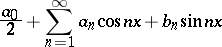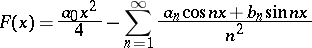# Riemann summation method

(diff) ← Older revision | Latest revision (diff) | Newer revision → (diff)

A method for summing series of numbers. A seriescan be summed by Riemann's method to a numberifThis method was first introduced and its regularity was first proved by B. Riemann in 1854 (see ). The Riemann summation method has been applied in the theory of trigonometric series, where it is usually stated as follows: A trigonometric serieswith bounded coefficientscan be summed by Riemann's method at a pointto a numberif the functionhas, at, Riemann derivative equal to.

How to Cite This Entry:
Riemann summation method. Encyclopedia of Mathematics. URL: http://encyclopediaofmath.org/index.php?title=Riemann_summation_method&oldid=17922
This article was adapted from an original article by T.P. Lykashenko (originator), which appeared in Encyclopedia of Mathematics - ISBN 1402006098. See original article